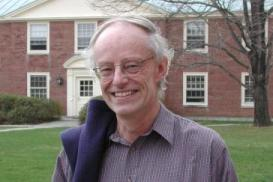# The collected works of James G. ArthurPhoto: Bill Casselman

James Arthur was born on May 18, 1944. He attended the University of Toronto as an undergraduate, and received his Ph.D. at Yale University in 1970, where his advisor was Robert Langlands. He has been a University Professor at the University of Toronto since 1987.

## The papers (arranged by topic)

### Unrefined trace formula and truncationThe Selberg trace formula for groups of F-rank one (1974)A truncation process for reductive groups (1977)Eisenstein series and the trace formula (1979)A trace formula for reductive groups I. Terms associated to classes in G(Q) (1978)A trace formula for reductive groups II. Applications of a truncation operator (1980)The trace formula for reductive groups (1983)

### Invariant trace formula and refined expansionsThe trace formula in invariant form (1981)On the inner product of truncated Eisenstein series (1982)On a family of distributions obtained from Eisenstein series I. Application of the Paley-Wiener theorem (1982)On a family of distributions obtained from Eisenstein series II. Explicit formulas (1982)The trace formula for noncompact quotient (1984)A measure on the unipotent variety (1985)On a family of distributions obtained from orbits (1986)The local behaviour of weighted orbital integrals (1988)The invariant trace formula I. Local theory (1988)The invariant trace formula II. Global theory (1988)

### Stable trace formula and endoscopyStability and endoscopy: informal motivation (1997)Towards a stable trace formula (1998)On the transfer of distributions: weighted orbital integrals (1999)Endoscopic L-functions and a combinatorial identity (1999)Stabilization of a family of differential equations (2000)A stable trace formula II. Global descent (2001)A stable trace formula I. General expansions (2002)A stable trace formula III. Proof of the main theorems (2003)Parabolic transfer for real groups (2008)The endoscopic classification of representations (2013)

### Characters, Hecke operators, L2-cohomologySome tempered distributions on semisimple groups of real rank one (1974)The characters of discrete series as orbital integrals (1976)The characters of supercuspidal representations as weighted orbital integrals (1987)Characters, harmonic analysis, and an L2-Lefschetz formula (1988)The trace formula and Hecke operators (1989)The L2-Lefschetz numbers of Hecke operators (1989)L2-cohomology and automorphic representations (1996)On local character relations (1996)

### Intertwining operatorsIntertwining operators and residues I. Weighted characters (1989)Intertwining operators and residues II. Invariant distributions (1989)Canonical normalization of weighted characters and a transfer conjecture (1998)Induced representations, intertwining operators, and transfer for real groups (2008)

### Harmonic analysis and nonabelian Fourier transformsHarmonic analysis of the Schwartz space of a reductive Lie group I,II, preprint (1974)A theorem on the Schwartz space of a reductive Lie group (1975)Harmonic analysis of invariant distributions (1978)A Paley-Wiener theorem for real reductive groups (1983)Multipliers and a Paley-Wiener theorem for real reductive groups (1983)The Fourier transform of weighted orbital integrals on SL(2,R) (1986)Harmonic analysis of tempered distributions on semisimple Lie groups of real rank one (1989)The trace Paley Wiener theorem for Schwartz functions (1994)An asymptotic formula for real groups (2006)Problems for real groups (2008)Germ expansions for real groups (2016)

### Local trace formula and applicationsTowards a local trace formula (1989)Some problems in local harmonic analysis (1991)A local trace formula (1991)On the Fourier transforms of weighted orbital integrals (1994)

### Functoriality and classification of representationsOn some problems suggested by the trace formula (1984)Unipotent automorphic representations: conjectures (1989)Unipotent automorphic representations: global motivation (1990)On elliptic tempered characters (1993)The problem of classifying automorphic representations of classical groups (1997)A note on the automorphic Langlands group (2002)The principle of functoriality (2003)Automorphic representations of GSp(4) (2004)A note on L-packets (2006)The embedded eigenvalue problem for classical groups (2008)Classifying automorphic representations (2012)

### Beyond EndoscopyProblems Beyond Endoscopy (2015)A stratification related to characteristic polynomials (2017)Functoriality and the Trace FormulaBeyond Endoscopy and elliptic terms (2017)

### MonographsSimple Algebras, Base Change, and the Advanced Theory of the Trace Formula (1989)The Endoscopic Classification of Representations: Orthogonal and Symplectic Groups. Published in 2013 as volume 61 in the series of Colloquium Publications by the American Mathematical Society.

### General expositionAutomorphic representations and number theory (1981)Lectures on automorphic L-functions (1991)Harmonic analysis and group representations (2000)An introduction to the Trace Formula (2005)Report on the trace formula (2009)On parameters for the group SO(2n) (to appear)Eigenfamilies, Characters and Multiplicities (to appear)L-functions and automorphic representations (2014)

### MiscellaneousArmand Borel (1923--2003) (2004)Harmonic analysis in mathematics (2005)AMS President's address at Abel celebration (2005)A (very brief) history of the trace formula (2007)Transfer, the Fundamental Lemma, and the work of Waldspurger (2009)The work of Ngô Bau Châu (2010)Memories of a Key Meeting (2017)Characters and modules (2019)